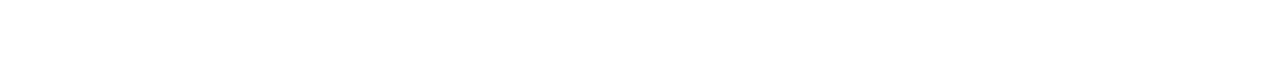# 15.2.1 Kinetic Theory of Gases

### Assumptions of the Kinetic Theory of Gases

• Gases consist of atoms or molecules randomly moving around at high speeds
• The kinetic theory of gases models the thermodynamic behaviour of gases by linking the microscopic properties of particles (mass and speed) to macroscopic properties of particles (pressure and volume)

• The theory is based on a set of the following assumptions:
• Molecules of gas behave as identical, hard, perfectly elastic spheres
• The volume of the molecules is negligible compared to the volume of the container
• The time of a collision is negligible compared to the time between collisions
• There are no forces of attraction or repulsion between the molecules
• The molecules are in continuous random motion
• The number of molecules of gas in a container is very large, therefore the average behaviour (eg. speed) is usually considered

#### Exam Tip

Make sure to memorise all the assumptions for your exams, as it is a common exam question to be asked to recall them.

### Root-Mean-Square Speed

• The pressure of an ideal gas equation includes the mean square speed of the particles:

<c2>

• Where
• c = average speed of the gas particles
• <c2> has the units m2 s-2
• Since particles travel in all directions in 3D space and velocity is a vector, some particles will have a negative direction and others a positive direction
• When there are a large number of particles, the total positive and negative velocity values will cancel out, giving a net zero value overall
• In order to find the pressure of the gas, the velocities must be squared
• This is a more useful method, since a negative or positive number squared is always positive
• To calculate the average speed of the particles in a gas, take the square root of the mean square speed:• cr.m.s is known as the root-mean-square speed and still has the units of m s-1
• The mean square speed is not the same as the mean speed### Author: Katie

Katie has always been passionate about the sciences, and completed a degree in Astrophysics at Sheffield University. She decided that she wanted to inspire other young people, so moved to Bristol to complete a PGCE in Secondary Science. She particularly loves creating fun and absorbing materials to help students achieve their exam potential.
CloseClose